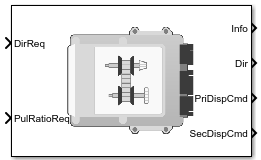CVT Controller

Continuously variable transmission controller

• Library:
• Powertrain Blockset / Transmission / Transmission Controllers

•Description

The CVT Controller block implements a push belt continuously variable transmission (CVT) controller. The block uses standard pulley and geometric equations to calculate the kinematic setpoints for the CVT variator. You can use the block to control a CVT.

Pulley Kinematics

Using the physical dimensions of the system, the block calculates the primary and secondary variator positions that meet the pulley ratio request.

The figure and equations summarize the geometric dependencies.$\begin{array}{l}{C}_{dist}=r{p}_{max}+{r}_{gap}+{r}_{sec_max}\\ {L}_{0}=f\left(r{p}_{max},r{s}_{max},r{p}_{min},r{s}_{min},{C}_{dist}\right)\\ rati{o}_{command}=f\left(rati{o}_{request},rati{o}_{max},rati{o}_{min}\right)\\ {r}_{pri}=f\left({r}_{0},rati{o}_{command},{C}_{dist}\right)\\ {r}_{\mathrm{sec}}=f\left({r}_{0},rati{o}_{command},{C}_{dist}\right)\\ {x}_{pri}=f\left({r}_{0},{r}_{pri},{\theta }_{wedge}\right)\\ {x}_{\mathrm{sec}}=f\left({r}_{0},{r}_{\mathrm{sec}},{\theta }_{wedge}\right)\end{array}$

The equations use these variables.

 ratiorequest Pulley gear ratio request ratiocommand Pulley gear ratio command, based on request and physical limitations rgap Gap distance between variator pulleys Cdist Distance between variator pulley centers rpmax Maximum variator primary pulley radius rsmax Maximum variator secondary pulley radius rpmin Minimum variator primary pulley radius rsmin Minimum variator secondary pulley radius ro Initial pulley radii with gear ratio of 1 Lo Initial belt length, resulting from variator specification xpri Variator primary pulley displacement, resulting from controller request xsec Variator secondary pulley displacement, resulting from controller request rpri Variator primary pulley radius, resulting from controller request rsec Variator secondary pulley radius, resulting from controller request Θwedge Variator wedge angle Φ Angle of belt to pulley contact point L Belt length, resulting from variator position

Ports

Inputs

expand all

Direction request, Dirreq, controlling the direction, either forward or reverse. Dir equals 1 for forward motion. Dir equals -1 for reverse.

CVT pulley ratio request, ratiorequest.

Output

expand all

Bus signal containing these block calculations.

SignalDescriptionVariableUnits

Variator primary pulley radius, resulting from controller request

rpri

m

Variator secondary pulley radius, resulting from controller request

rsec

m

Initial pulley radii with gear ratio of 1

ro

m

Pulley gear ratio command, based on request and physical limitations

ratiocommand

N/A

RatioMax

Maximum pulley ratio

ratiomax

N/A

RatioMin

Minimum pulley ratio

ratiomin

N/A

PriDispCmd

Variator primary pulley displacement, resulting from controller request

xpri

m

SecDispCmd

Variator secondary pulley displacement, resulting from controller request

xsec

m

Direction request, Dirreq, controlling the direction, either forward or reverse. Dir equals 1 for forward motion. Dir equals -1 for reverse.

Variator primary pulley displacement, xpri, in m.

Variator secondary pulley displacement, xsec, in m.

Parameters

expand all

Kinematics

Maximum variator primary pulley radius, rpmax, in m.

Maximum variator secondary pulley radius, rsmax, in m.

Minimum variator primary pulley radius, rpmin, in m.

Minimum variator secondary pulley radius, rsmin, in m.

The gap between the secondary and primary pulleys, rgap, in m. The figure shows the pulley geometry.Variator wedge angle, Θwedge, in deg.Ambekar, Ashok G. Mechanism and Machine Theory. New Delhi: Prentice-Hall of India, 2007.

 Bonsen, B. Efficiency optimization of the push-belt CVT by variator slip control. Ph.D. Thesis. Eindhoven University of Technology, 2006.

 CVT How Does It Work. CVT New Zealand 2010 Ltd. February 10, 2011. Accessed April 25, 2016.

 Klaassen, T. W. G. L. The Empact CVT: Dynamics and Control of an Electromechanically Actuated CVT. Ph.D. Thesis. Eindhoven University of Technology, 2007.

Powertrain Blockset DocumentationGet trial now